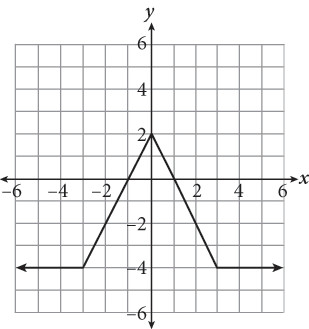# SAT Math Multiple Choice Question 333: Answer and Explanation

### Test Information

Question: 333

3.The figure above shows the graph of f(x). For which value(s) of x does f(x) equal 0?

• A. –3 and 3
• B. –1 and 1
• C. –1, 1, and 2
• D. 2 only